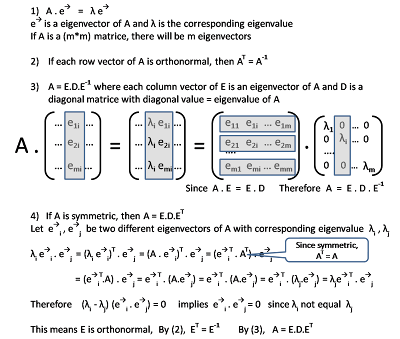## Sunday, November 1, 2009

### Principal Component Analysis

One common problem of machine learning is the "curse of high dimensionality". When there are too many attributes in the input data, many of the ML algorithms will be very inefficient or some of them will even be non-performing (e.g. in nearest neighbor computation, data points in a high-dimensional space are pretty much equal distance with each other).

It is quite possible that the attributes we selected are inter-dependent on each other. If so, we may be able to extract a smaller subset of independent attributes that may still be very useful to describe the data characteristics. In other words, we may be able to reduce the number of dimensions significantly without losing much fidelity of the data.

"Dimension Reduction" is a technique to determine how we can reduce the number of dimensions while minimizing the loss of fidelity of data characteristics. It is typically applied during the data cleansing stage before feeding into the machine learning algorithm.

"Feature Selection" is a simple techniques to select a subset of features that is more significant. A very simple "filtering" approach can be used by looking at each attribute independently and rank their significance using some measurement (e.g. info gain) and throw away those that has minimum significance. A more sophisticated "wrapper" approach is to look at different subset of features to do the evaluation. There are two common model in the "wrapper" approach, "forward selection" and "backward elimination".

In forward selection, we start with no attribute and then start to pick the attribute with the highest statistical significance, (ie: prediction improves a lot from the cross validation check). After picking the first attribute, and we start to pair it up with another unselected attribute and find the one with the most significant improvement in the cross-validation-check. We keep growing the set of attributes until we don't find significant improvements. One issue of the "forward selection" approach is that it may miss "grouped features". For example, attribute1 and attribute2 may be insignificant when they are stand-alone, but combining them will give very big improvement.

Backward elimination can be used to handle this problem. It basically goes the opposite direction, starts with the full set of attributes and start to drop those attribute that has least statistical significance (ie: prediction degrades very little from the cross validation check). The downside of "backward elimination" is that it is much more expensive to run.

A more powerful approach called "Feature Extraction" is more commonly used to extract a different set of attributes by linearly combining the existing set of attributes. Principal Component Analysis "PCA" is a very popular technique in this arena. PCA can analyze the interdependency between pair of attributes and identify those significant ones.

The intuition of PCA
The intuition is to rearrange the linear combination of existing m attributes in different way to form another set of m attributes. The new set of attributes has the characteristic that
• Each attribute is independent of each other
• The set of attributes is ranked according to the range of variation
Note that attributes with narrow range of variation doesn't provide much information to describe the data samples and so can be ignored with minimal lost of fidelity. So we remove that to reduce the dimensionality.

The question is : How do we recompose the m attributes to exhibit the above 2 characteristics. Lets take a deeper look into it.

Underlying theory of PCA
Assume there are N data points in the input data set and each data point is described by M attributes. We use the statistical definition for the "mean", "variance" of each attribute and "co-variance" for every pair of attributes. Co-variance is an indicator of dependencies of two attributes with zero implies independence.In an ideal situation, we want COV-x to be a diagonal matrix, which means COV(i, j) to be zero. In other words, all pairs of attribute-i and attribute-j are independent to each other. We also want the diagonal to be ranked in descending order.

So the problem can be reduced to finding a different combination of the m attributes to form a new set of m attributes (Y = P. X) such that COV-y is a ranked diagonal matrix.

How do we determine P ?Some Matrix theory
Here is a review of Matrice theory that will be usedLets find the transformation matrice PSo the PCA process can be summarized in following ...
1. Input: X, a matrice of (m * n), a set of N sample data points, each with M attributes.
2. Compute Cov-X, a matrice of (m * m), the Covariance matrice of X
3. Compute the m Eigenvectors and m Eigenvalues of Cov-X
4. Order the Eigenvectors according to the Eigenvalues
5. Now found the transformation matrice P, which is a matrice of (m * m). Note that each row vector of P corresponding to an eigenvector, which is effectively the axis of the new co-ordinate system.
6. Truncate P to just take the top k rows. Now P' is a (k * m) matrice.
7. Apply P' . X to all input data to result in a matrice of (k * n). This is effectively reduce each data point from m-dimension to k-dimension.

References
A very good paper
Some Matrix math review and step by step PCA calculationAnonymous said...

Dear Sir,

Can you please show an working example of PCA in a step-wise (with sample data and/or free software used to achieve this), way so that I can use the same for my data.

Kindly, help me.

Thanking you,
Siva

Jeyachandran said...

dear sir

am working PCA in image processing ,finally i found the concept of PCA but i cant understand the physical interpretation in matrix operation.